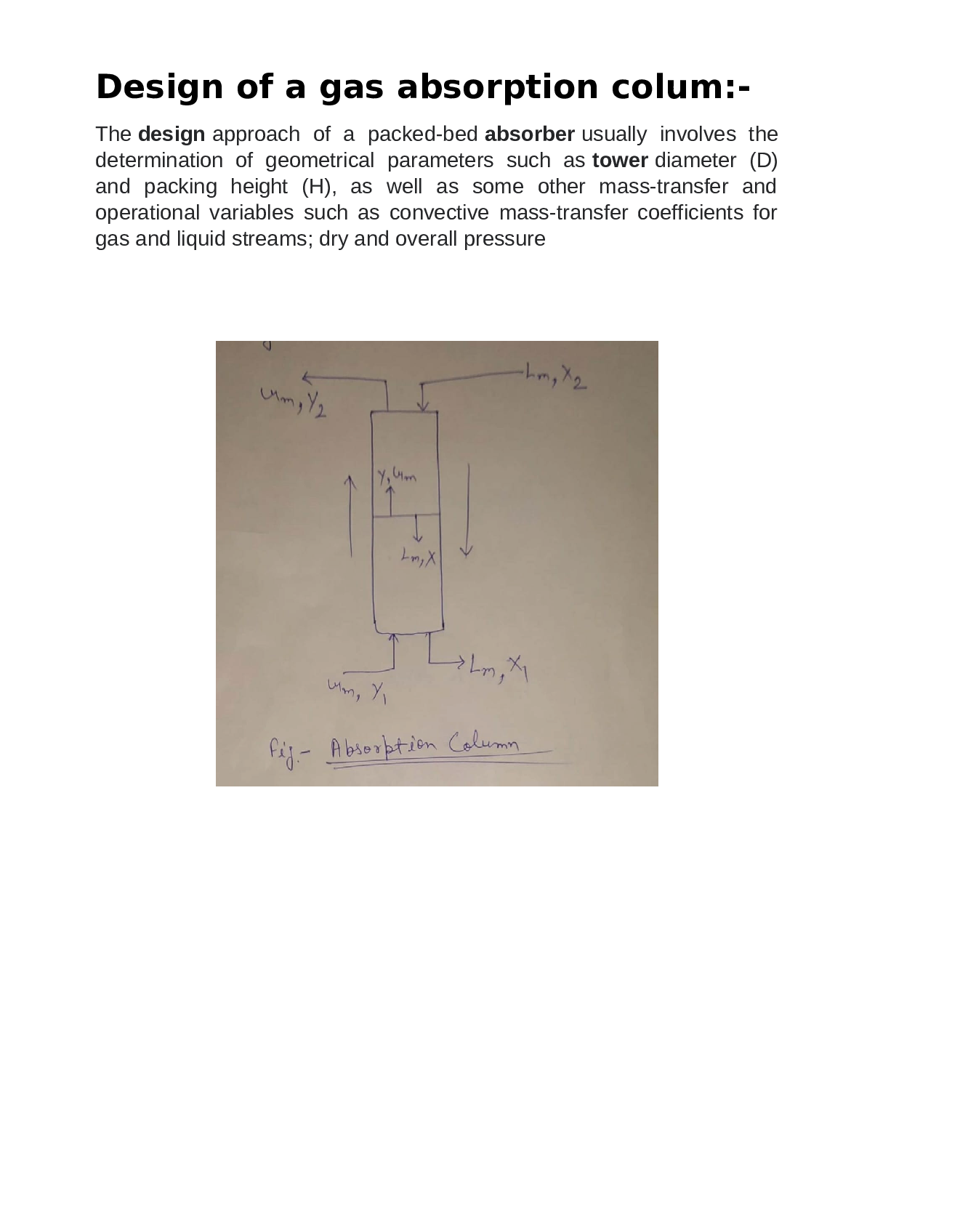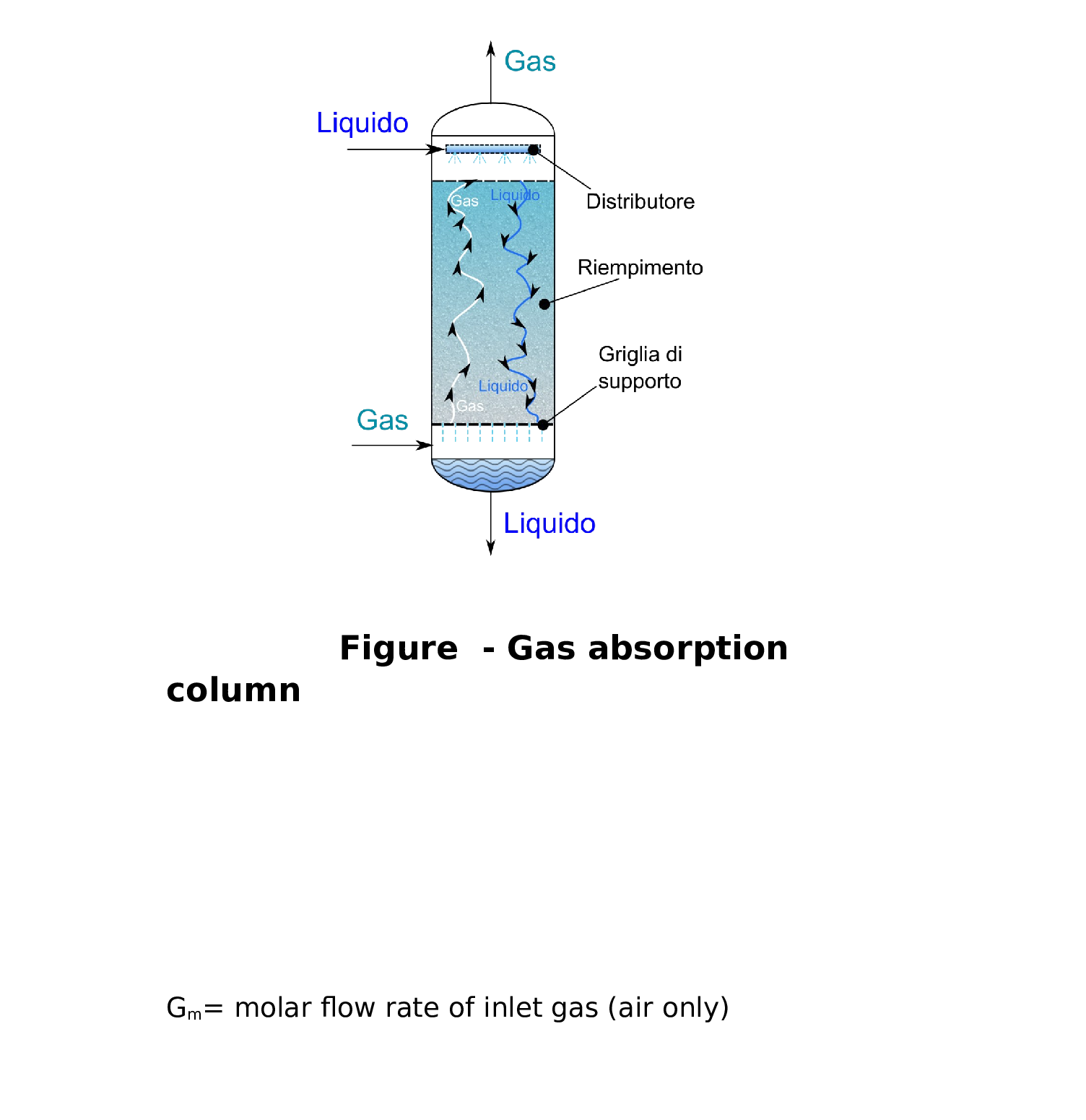Question

Chemical Thermodynamics

A gas stream containing 3 mol% Ammonia (NH3) in Air is to be passed to a packed absorption columnat a rate of 5 kg s1. The column is to use Water (H20) as the solvent to reduce the ammonia content in the air leaving the column to 0.01 mol%. The gas and water streams can be assumed to be at 25 °C.The column operates at 1 bar pressure.

y = Hx

What the liquid rate of solvent is under normal operating conditions.

Where the Henry's Law constant, H = 1.3 (mole frac NH3 in gas) (mole frac NH3 in liquid)1

From pilot scale experiments the Overall Mass Transfer Coefficient, KG, has been found to remain constant with a value of 200 × 10--6 kmol m² s-1

Using the protocol outlined on Page 55 of the gas absorption notes, specify an absorption column to achieve the required separation using 1 inch Raschig Rings (See Table 1 in attached data sheets).

Your Design Specification must clearly show the following:

Any assumptions made must be stated clearly.

What the minimum liquid rate for the column is.

Confirmation that the wetting rate of your column falls within the acceptable range.

Based on the assumption of operating at 60% of the flooding gas flow rate, what the diameter of the column should be in meters.

The values of HTU, NTU and thus the total height of packing required in the column.

The relationship that describes the equilibrium between Ammonia and Water at these conditions is given by:Verified### Question 45710Chemical Thermodynamics

A. How do the following factors affect the spontaneity of a reaction? (2 points)
i. Enthalpy (1 point)
ii. Entropy (1 point)
B. What is the equation that relates these factors? What is it called? (1 point)
C. How is Gibbs free energy used to predict the spontaneity of a reaction? (1 point)

### Question 45709Chemical Thermodynamics

A. What does the second law of thermodynamics say about entropy? (1 point)
B. Which phase has the lowest entropy? Why? How does a change in phase affect entropychanges in a reaction? (2 points)
C. How do reactions involving gases affect the entropy of a system? (1 point)

### Question 45708Chemical Thermodynamics

A. Explain the difference between an exothermic chemical reaction and an endothermicchemical reaction. (1 point)
B. How is the enthalpy of reaction related to the enthalpies of formation for the products and reactants? (1 point)
C. What is Hess's law? (1 point)
D. How does Hess's law use intermediate reactions to calculate the enthalpy of a desired reaction? (2 points)
E. Mark each description as an exothermic or endothermic reaction. (2 points)

### Question 45707Chemical Thermodynamics

A. Describe the following heat equations, and identify the indicated variables. (3 points)
\text { i. } Q=m c \Delta T \text {; identify c. (1 point) }
Q=m L_{\text {vapori }} \text { identify } L_{\text {vapor }}
\text { i. } Q=m L_{\text {fusion }} \text {; identify } L_{\text {fusion }} \text {. }
B. Describe the temperature changes that occur in ice as energy is added, starting in the frozen state and ending in the vapor state. (2 points)

### Question 45706Chemical Thermodynamics

A. What is the first law of thermodynamics? (1 point)
B. Match each term to its description or definition. (4 points)
А. Нeat
B. Thermal energy
C. Conduction
D. Convection
F. Specific heat
G. Enthalpy
H. Entropy
A measure of the randomness in a system
Heat transfer through direct contact between molecules
Heat required to raise 1 g of a substance 1°C
The transfer of thermal energy between objects
Heat given off or absorbed in a reaction
Heat transfer by way of electromagnetic
Total kinetic energy of all molecules in asystem
Heat transfer through movement of liquids or gases

### Question 45262Chemical Thermodynamics

Problem 5 a) Calculate the equilibrium constant at 1000 °C for ammonia synthesis in the stoichiometry written below:
3H2 + N2 = 2NH3
b) An equimolar mixture of the three species enters a reactor at 1000°C, 10 bar. Determine the compositionat equilibrium, if temperature and pressure remain constant.
c) Determine the amount of heat that must be added to, or removed from the reactor to maintain the temperature at 1000°C. Report the result in J per mol of hydrogen in the feed.
Additional data: The ideal gas Cp (in J/mol K) of hydrogen, nitrogen, and ammonia are 29.52, 30.58, and45.4, respectively.

### Question 45261Chemical Thermodynamics

Problem 4 a) Determine the heat of vaporization of benzene at 25 °C using tabulated heats of formation.
b) Determine Henry's law constant of ammonia in water at 25 °C from the tabulated (g) and (ag) Gibbs energies of formation.
c) Generate an entry for the Gibbs energy of formation of CO based on the standard state for aqueous solutes
d) N2O gas is bubbled through water at 12 bar, 10 °C. Calculate the activity of N2O (aq) and of water (1) in the liquid based on the indicated standard states.

### Question 45260Chemical Thermodynamics

Problem 3 a) Calculate the standard heat of reaction for the complete combustion of methane at 800 °C.b) Calculate the amount of heat that is released in a furnace that burns methane in 20% excess air, if the furnace temperature is 800 °C at the pressure is 2 bar. Assume that the inlet gases are already at 800°C asthe enter the furnace.
3 a) Calculate the standard heat of reaction for the complete combustion of methane at 800 °C.
b) Calculate the amount of heat that is released in a furnace that burns methane in 20% excess air, if the furnace temperature is 800 °C at the pressure is 2 bar. Assume that the inlet gases are already at 800°C asthe enter the furnace.
c) Repeat part b but this time the inlet gases are at 40°C, 2 bar.
Additional data: Assume that the reaction is complete and that products are carbon dioxide and water.The heat capacities of the gases are given in Problem ??.

### Question 45259Chemical Thermodynamics

Problem 2 Methane is mixed with 20% excess air at 1 bar, 25°C, and the mixture is ignited. Assuming complete oxidation to carbon dioxide and water, calculate the adiabatic temperature in the following tow cases:
a) Assume the heat capacities of all species to be constant and equal to their value at 25 °C.
b) Assume the heat capacities to be functions of temperature.

### Question 45258Chemical Thermodynamics

Problem 1 A reactor is loaded with equal amounts of hydrogen, nitrogen, ammonia and helium. if thereaction is
3 \mathrm{H}_{2}+\mathbf{N}_{2}=2 \mathrm{NH}_{3}
a) Calculate the minimum and maximum values of .
b) Calculate all mole fractions when the mole fraction of helium is 0.3. What is the conversion of hydrogen?
c) Calculate all mole fractions when the mole fraction of helium is 0.215. What is the conversion of hydro-gen?

### Submit query